Chemguide: Support for CIE A level Chemistry ``` ``` Learning outcome 28: Chemistry of transition elements 28.2: General characteristic chemical properties of the first set of transition elements, titanium to copper ``` ``` Learning outcome 28.2.8 This statement is about relating E° values to the redox reactions involving transition metal compounds. Before you go on, you should find and read the statement in your copy of the syllabus. ``` ``` It is completely pointless going any further with this unless you are reasonably confident about the use of electrode potentials in deciding whether a reaction is feasible or not. That was all dealt with in this page in Section 24.2. You need to read the paragraph about statement 24.2.5. If you have forgotten all of this, you may well need to re-read the whole lot! I will look at a series of examples to show you the easiest way of thinking about this. ``` ``` Example 1 Explain why acidified potassium manganate(VII) solution can't be used in a titration involving iron(II) chloride solution, whereas acidified potassium dichromate(VI) can be used in the presence of chloride ions. The relevant E° values are: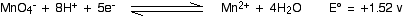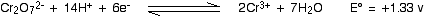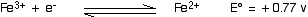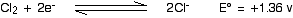``` ``` The reaction involving potassium manganate(VII) So what is the problem with potassium manganate(VII) in the presence of iron(II) chloride? Will it oxidise the iron(II) ions? Yes, it will. Remember that when you couple two of these equilibria together, the more positive one moves to the right, and the more negative (or less positive) moves to the left. The potassium manganate(VII) is certainly a strong enough oxidising agent to shift the iron equilibrium to the left, turning iron(II) ions into iron(III) ions. Its E° value is more positive than the iron one. So the problem must lie with the chloride ions. The E° value for the manganate(VII) equilibrium is more positive than the E° value for the chlorine equilibrium. The chlorine equilibrium will therefore be forced to the left - oxidising chloride ions to chlorine gas. That means that when you do the titration, the manganate(VII) ions are reacting with both the iron(II) ions and the chloride ions. You can't do a simple calculation from two separate reactions going on at the same time. ``` ``` The reaction involving potassium dichromate(VI) The dichromate E° is more positive than the iron one, so there won't be any problem oxidising the iron(II) ions to iron(III). The iron equilibrium will be driven to the left by the more positive dichromate one. However, the dichromate E° value isn't quite as positive as the chlorine one. Of the two equilibria, the chlorine one has a slightly greater tendency to move to the right. But if you start with chloride ions, it can't go any further right, and so there is no reaction. With acidified potassium dichromate(VI) solution, the iron(II) ions get oxidised, but nothing happens to the chloride ions - at least, as long as the chloride ion concentration isn't too great . . . ``` ``` Example 2 Why can potassium dichromate(VI) solution oxidise concentrated hydrochloric acid to chlorine? The E° values are:As we have just seen, there shouldn't be any reaction between dichromate ions and chloride ions - but obviously there is in this case. You have to remember that E° values only apply under standard conditions, and one of those conditions is that the ion concentrations are all 1 mol dm-3. But in concentrated hydrochloric acid, the ion concentrations are about 10 mol dm-3. That concentration change alters the positions of the two equilibria. In the dichromate equilibrium, the extra concentration of the hydrogen ions moves the position of equilibrium to the right (Le Chatelier's Principle). The electrode potential is a measure of the position of equilibrium, and so that makes the electrode potential more positive under these circumstances. Similarly, the higher concentration of chloride ions moves the position of the chlorine equilibrium to the left - making the electrode potential less positive. The two E° values were originally so close, that it wouldn't take much movement for their order to reverse - for the dichromate electrode potential to become more positive than the chlorine one. When that happens, the chlorine equilibrium will get driven to the left, and the potassium dichromate(VI) solution will oxidise chloride ions to chlorine. ``` ``` Example 3 Will tin(II) ions reduce iron(III) ions to iron(II) ions? The E° values are: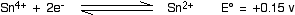The iron equilibrium is the more positive, and so will go in the forward direction. The tin equilibrium is less positive (more negative), and will be driven backwards. So the tin(II) ions will reduce iron(III) ions to iron(II) ions. In the process, of course, the tin(II) ions will be oxidised to tin(IV) ions. ``` ``` Example 4 If you add zinc to sodium vanadate(V) solution, it is reduced in a number of steps eventually to vanadium(II) ions. Sodium vanadate(V) solution can contain either VO3- or VO2+ ions depending on the conditions. I am going to give you the equations starting from VO3-, because it is less confusing. If you possibly can, look at a short bit of video on YouTube, showing what happens if you shake sodium vanadate(V) solution with zinc over several minutes. (The video condenses this down to about 1 minute in all.) Here are the E° values for the three changes you may have seen: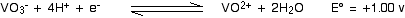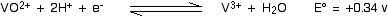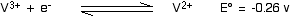In each case, the E° value for the zinc (-0.76 v) was more negative than all of these values. That allowed all these reactions to go to the right, and gave the colour changes. The original vanadate(V) ion was yellow. At the completion of the first change, the solution was blue. At the completion of the second change, the solution was green. At the completion of the final change, the solution was lavender-coloured. Important:  You DO NOT need to learn any of this for CIE. If they ask you a question like the one just coming up, they will give you all the information you need. So now the question . . . What colour would the solution end up if you used tin rather than zinc? The E° value for tin is: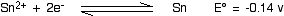For any of the vanadium reactions to move to the right, they have to be coupled with something with a more negative E° value. The tin E° value is more negative than either of the first two reactions, so those will go to the right, and the tin will go to the left producing tin(II) ions. However, in the last reaction, the vanadium equilibrium has the more negative E° value. That would be the one that would tend to move to the left - but that's where it is already. No further reaction is possible. So the tin will only reduce the vanadium(V) ions as far as the end of the second reaction, producing vanadium(III) ions. These are green. ``` ``` It is obviously impossible to think of every single example that CIE might use, but if you have followed the examples above without too much difficulty, you should be able to work out, or explain, what might happen in most cases. The only problem is that the person who sets the questions for CIE on E° values has a fixation about asking you to calculate overall cell potentials. If you aren't happy about those, you need to go and revise Section 24.2 - looking particularly at the section headed "Statements 24.2.1(b), 24.2.4 and 24.2.5". And in that part of the page, concentrate on the section headed "Calculating the cell potential from a given equation". ``` ``` Go to the Section 28 Menu . . . To return to the list of learning outcomes in Section 28 Go to the CIE Main Menu . . . To return to the list of all the CIE sections Go to Chemguide Main Menu . . . This will take you to the main part of Chemguide. © Jim Clark 2020